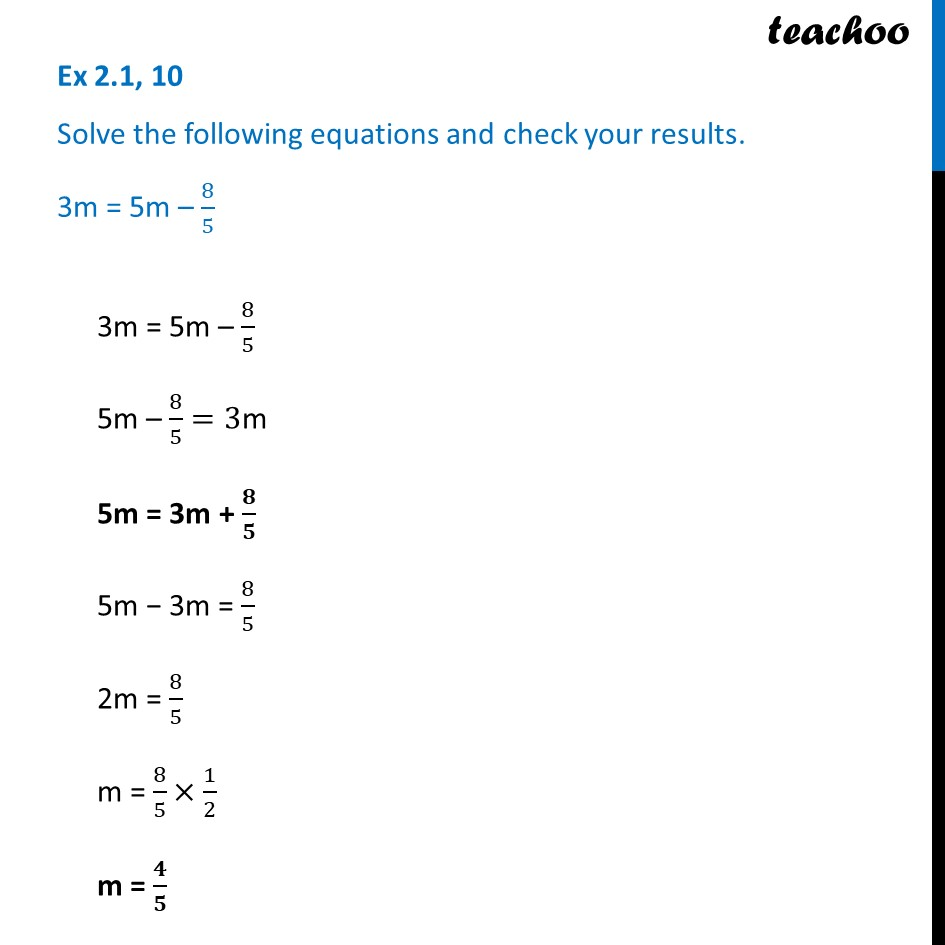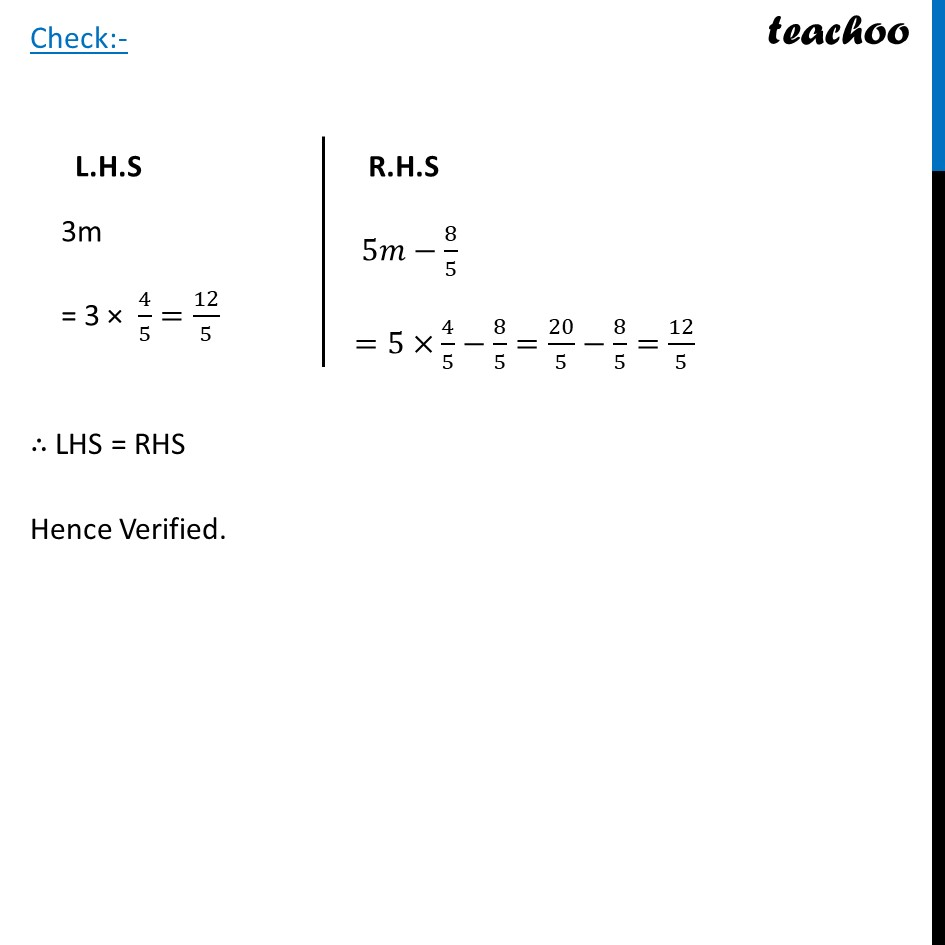Ex 2.1

Chapter 2 Class 8 Linear Equations in One Variable
Serial order wiseLearn in your speed, with individual attention - Teachoo Maths 1-on-1 Class

### Transcript

Ex 2.1, 10 Solve the following equations and check your results. 3m = 5m – 8/53m = 5m – 8/5 5m – 8/5=3m 5m = 3m + 𝟖/𝟓 5m − 3m = 8/5 2m = 8/5 m = 8/5×1/2 m = 𝟒/𝟓 L.H.S 3m = 3 × 4/5=12/5 R.H.S 5𝑚−8/5 =5×4/5−8/5=20/5−8/5=12/5 Check:- ∴ LHS = RHS Hence Verified.